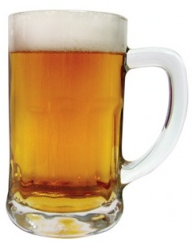﻿ Alcohol Metabolism Calculator ﻿# Alcohol Metabolism CalculatorTime to Metabolize Alcohol=
Blood Alcohol Level/20mg/dl

mg/dL

.

The Alcohol Metabolism Calculator calculates the amount of time that it takes for a person to metabolize a volume of alcohol.

The time is computed in units hours, how many hours it takes to metabolize the alcohol within the system.

The only input that a user must enter is the blood alcohol level (measured in mg/dL) that the person has. Blodo alcohol level is usually expressed as milligrams per deciliter- the amount of milligrams of alcohol that that are you in a tenth of a liter (a deciliter).

Based on this value, it can be computed how long it will take for the person to metabolize that alcohol.

Of course people have different metabolisms in terms of how fast it takes them to metabolize alcohol, but, on average, a person normally can metabolize 20mg/dL of alcohol in an hour's time.

Therefore, for example, if a person has a blood alcohol level of 180mg/dL, it will take (180mg/dL)/(20mg/dL)= 9 hours for a person to metabolize all of the alcohol in his or her system.

This alcohol metabolism calculation is good for knowing the amount of time that it takes for a certain amount of alcohol to be metabolized.

If you are creating an electronic device that measures blood alcohol levels, then this formula gives you a reference for knowing how much alcohol a person should have in their system after a certain amount of time has passed, assuming you know that the starting base blood alcohol level, you can have an approximation of what the person's blood alcohol level would be after a certain amount of time has passed.

Related Resources

﻿